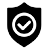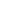#### WhatsApp Quick Chat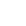# UPSC MAIN MATHEMATICS

#### Offers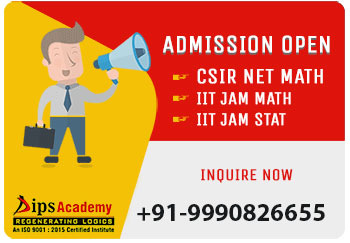##### News & Events# IAS Mains Mathematics Syllabus

#### IAS Mains Mathematics Syllabus

##### Paper-I

Linear Algebra: Vector spaces over R and C, linear dependence and independence, subspaces, bases, dimension; Linear transformations, rank and nullity, matrix of a linear transformation.
Algebra of Matrices; Row and column reduction, Echelon form, congruence’s and similarity; Rank of a matrix; Inverse of a matrix; Solution of system of linear equations; Eigenvalues and eigenvectors, characteristic polynomial, Cayley-Hamilton theorem, Symmetric, skew-symmetric, Hermitian, skew-Hermitian, orthogonal and unitary matrices and their eigenvalues.

Calculus: Real numbers, functions of a real variable, limits, continuity, differentiability, mean-value theorem, Taylor's theorem with remainders, indeterminate forms, maxima and minima, asymptotes; Curve tracing; Functions of two or three variables: limits, continuity, partial derivatives, maxima and minima, Lagrange's method of multipliers, Jacobian.
Riemann's definition of definite integrals; Indefinite integrals; Infinite and improper integrals; Double and triple integrals(evaluation techniques only); Areas, surface and volumes.

Analytic Geometry: Cartesian and polar coordinates in three dimensions, second degree equations in three variables, reduction to canonical forms, straight lines, shortest distance between two skew lines; Plane, sphere, cone, cylinder, paraboloid, ellipsoid, hyperboloid of one and two sheets and their properties.

Ordinary Differential Equations: Formulation of differential equations; Equations of first order and first degree, integrating factor; Orthogonal trajectory; Equations of first order but not of first degree, Clairaut's equation, singular solution. Second and higher order linear equations with constant coefficients, complementary function, particular integral and general solution. Second order linear equations with variable coefficients, Euler-Cauchy equation; Determination of complete solution when one solution is known using method of variation of parameters.

Laplace and Inverse Laplace transforms and their properties; Laplace transforms of elementary functions. Application to initial value problems for 2nd order linear equations with constant coefficients.

Dynamics & Statics: Rectilinear motion, simple harmonic motion, motion in a plane, projectiles; constrained motion; Work and energy, conservation of energy; Kepler's laws, orbits under central forces.

Equilibrium of a system of particles; Work and potential energy, friction; common catenary; Principle of virtual work; Stability of equilibrium, equilibrium of forces in three dimensions.

Vector Analysis: Scalar and vector fields, differentiation of vector field of a scalar variable; Gradient, divergence and curl in cartesian and cylindrical coordinates; Higher order derivatives; Vector identities and vector equations. Application to geometry: Curves in space, Curvature and torsion; Serret-Frenet’s formulae. Gauss and Stokes’ theorems, Green’s identities.

##### Paper-II

(1) Algebra: Groups, subgroups, cyclic groups, cosets, Lagrange’s Theorem, normal subgroups, quotient groups, homomorphism of groups, basic isomorphism theorems, permutation groups, Cayley’s theorem.
Rings, subrings and ideals, homomorphisms of rings; Integral domains, principal ideal domains, Euclidean domains and unique factorization domains; Fields, quotient fields.

Real Analysis: Real number system as an ordered field with least upper bound property; Sequences, limit of a sequence, Cauchy sequence, completeness of real line; Series and its convergence, absolute and conditional convergence of series of real and complex terms, rearrangement of series.

Continuity and uniform continuity of functions, properties of continuous functions on compact sets.

Riemann integral, improper integrals; Fundamental theorems of integral Calculus.
Uniform convergence, continuity, differentiability and integrability for sequences and series of functions; Partial derivatives of functions of several (two or three) variables, maxima and minima.

Complex Analysis: Analytic functions, Cauchy-Riemann equations, Cauchy's theorem, Cauchy's integral formula, power series representation of an analytic function, Taylor’s series; Singularities; Laurent's series; Cauchy's residue theorem; Contour integration.

Linear Programming: Linear programming problems, basic solution, basic feasible solution and optimal solution; Graphical method and simplex method of solutions; Duality.
Transportation and assignment problems.

Partial differential equations: Family of surfaces in three dimensions and formulation of partial differential equations; Solution of quasilinear partial differential equations of the first order, Cauchy's method of characteristics; Linear partial differential equations of the second order with constant coefficients, canonical form; Equation of a vibrating string, heat equation, Laplace equation and their solutions.

Numerical Analysis and Computer programming: Numerical methods: Solution of algebraic and transcendental equations of one variable by bisection, Regula-Falsi and Newton-Raphson methods; solution of system of linear equations by Gaussian elimination and Gauss-Jordan (direct), Gauss-Seidel(iterative) methods. Newton's (forward and backward) interpolation, Lagrange's interpolation.

Numerical integration: Trapezoidal rule, Simpson's rules, Gaussian quadrature formula.
Numerical solution of ordinary differential equations: Euler and Runga Kutta-methods.
Computer Programming: Binary system; Arithmetic and logical operations on numbers; Octal and Hexadecimal systems; Conversion to and from decimal systems; Algebra of binary numbers.
Elements of computer systems and concept of memory; Basic logic gates and truth tables, Boolean algebra, normal forms.
Representation of unsigned integers, signed integers and reals, double precision reals and long integers.
Algorithms and flow charts for solving numerical analysis problems.

Mechanics and Fluid Dynamics: Generalized coordinates; D' Alembert's principle and Lagrange's equations; Hamilton equations; Moment of inertia; Motion of rigid bodies in two dimensions.
Equation of continuity; Euler's equation of motion for inviscid flow; Stream-lines, path of a particle; Potential flow; Two-dimensional and axisymmetric motion; Sources and sinks, vortex motion; Navier-Stokes equation for a viscous fluid.

## Offers## News & Events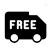##### Free Delivery